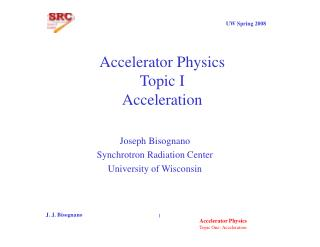# Accelerator Physics Topic I Acceleration - PowerPoint PPT PresentationDownload PresentationAccelerator Physics Topic I Acceleration

Accelerator Physics Topic I Acceleration
Download Presentation## Accelerator Physics Topic I Acceleration

- - - - - - - - - - - - - - - - - - - - - - - - - - - E N D - - - - - - - - - - - - - - - - - - - - - - - - - - -
##### Presentation Transcript

1. Accelerator PhysicsTopic IAcceleration Joseph Bisognano Synchrotron Radiation Center University of Wisconsin J. J. Bisognano

2. Relativity J. J. Bisognano

3. Maxwell’s Equations J. J. Bisognano

4. Vector Identity Games Poynting Vector Electromagnetic Energy J. J. Bisognano

5. Propagation in Conductors J. J. Bisognano

6. Free Space Propagation J. J. Bisognano

7. Conductive Propagation J. J. Bisognano

8. Boundary Conditions J. J. Bisognano

9. AC Resistance J. J. Bisognano

10. Cylindrical Waveguides • Assume a cylindrical system with axis z • For the electric field we have • And likewise for the magnetic field J. J. Bisognano

11. Solving for Etangential J. J. Bisognano

12. Maxwell’s equations then imply (k=/c) J. J. Bisognano

13. All this implies that E0zandB0z tell it all with their equations • For simple waveguides, there are separate solutions with one or other zero (TM or TE) • For complicated geometries (periodic structures, dielectric boundaries), can be hybrid modes J. J. Bisognano

14. TE Rectangular Waveguide Mode x a y b J. J. Bisognano

15. a TE mode Example J. J. Bisognano

16. Circular Waveguide TEm,nModes J. J. Bisognano

17. Circular Waveguide TEm,nModes J. J. Bisognano

18. Circular Waveguide TMm,nModes J. J. Bisognano

19. Circular Waveguide Modes J. J. Bisognano

20. Cavities d J. J. Bisognano

21. Cavity Perturbations Now following C.C. Johnson, Field and Wave Dynamics J. J. Bisognano

22. Cavity Energy and Frequency I ++++ E B - - - - -I Attracts Repels J. J. Bisognano

23. Energy Change of Wall Movement J. J. Bisognano

24. Bead Pull J. Byrd J. J. Bisognano

25. Lorentz Theorem • Let and be two distinct solutions to Maxwell’s equations, but at the same frequency • Start with the expression J. J. Bisognano

26. Vector Arithmetic J. J. Bisognano

27. Using curl relations for non-tensor m, e one can show that expression is zero • So, in particular, for waveguide junctions with an isotropic medium we have S2 S3 S1 J. J. Bisognano

28. Scattering Matrix • Consider a multiport device • Discussion follows Altman S2 Sp S1 J. J. Bisognano

29. S-matrix • Let apamplitude of incident electric field normalize so that ap2 = 2(incident power) and bp2 = 2(scattered power) J. J. Bisognano

30. Two-Port Junction Port X Port Y a2 a1 b2 b1 J. J. Bisognano

31. Implication of Lorentz Theorem J. J. Bisognano

32. Lorentz/cont. • Lorentz theorem implies • or J. J. Bisognano

33. Unitarity of S-matrix • Dissipated power P is given by • For a lossless junction and arbitrary this implies J. J. Bisognano

34. Symmetrical Two-Port Junction J. J. Bisognano

35. Powering a Cavity b1 b2 a1 a2  J. J. Bisognano

36. Power Flow J. J. Bisognano

37. Power Flow/cont. J. J. Bisognano

38. Optimization • With no beam, best circumstance is ; I.e., no reflected power J. J. Bisognano

39. At Resonance J. J. Bisognano

40. Shunt Impedance • Consider a cavity with a longitudinal electric field along the particle trajectory • Following P. Wilson z2 z1 J. J. Bisognano

41. Shunt Impedance/cont J. J. Bisognano

42. Shunt Impedance/cont. • Define • where P is the power dissipated in the wall (the term) • From the analysis of the coupling “b” • where is the generator power J. J. Bisognano

43. Beam Loading • When a point charge is accelerated by a cavity, the loss of cavity field energy can be described by a charge induced field partially canceling the existing field • By superposition, when a point charge crosses an empty cavity, a beam induced voltage appears • To fully describe acceleration, we need to include this voltage • Consider a cavity with an excitation V and a stored energy • What is ? J. J. Bisognano

44. Beam Loading/cont. • Let a charge pass through the cavity inducing and experiencing on itself. • Assume a relative phase • Let charge be bend around for another pass after a phase delay of  J. J. Bisognano

45. Beam Loading/cont. Ve e V2 q V1 +V2 V1 J. J. Bisognano

46. Beam Loading/cont. • With negligible loss • But particle loses • Since q is arbitrary, e =0 and J. J. Bisognano

47. Beam Loading/cont. • Note: we have same constant (R/Q) determining both required power and charge-cavity coupling J. J. Bisognano

48. Beam Induced Voltage • Consider a sequence of particles at J. J. Bisognano

49. Summary of Beam Loading • References: Microwave Circuits (Altman); HE Electron Linacs (Wilson, 1981 Fermilab Summer School) J. J. Bisognano

50. Vector Addition of RF Voltages Vb Vc Vg y j Vgr Vbr y q Vb J. J. Bisognano